# 2. 示例：绘制三角形

## 1) HelloTriangle.html

<!DOCTYPE html>
<html lang="en">
<meta charset="utf-8" />
<title>Hello Triangle</title>

<canvas id="webgl" width="400" height="400">
Please use a browser that supports "canvas"
</canvas>

<script src="../lib/webgl-utils.js"></script>
<script src="../lib/webgl-debug.js"></script>
<script src="../lib/cuon-utils.js"></script>
<script src="HelloTriangle.js"></script>
</body>
</html>


## 2) HelloTriangle.js

// 顶点着色器程序
'attribute vec4 a_Position;\n' + // attribute variable
'void main() {\n' +
'  gl_Position = a_Position;\n' + // Set the vertex coordinates of the point
'}\n';

// 片元着色器程序
'precision mediump float;\n' +
'uniform vec4 u_FragColor;\n' +  // uniform変数
'void main() {\n' +
'  gl_FragColor = vec4(1.0, 0.0, 0.0, 1.0);\n' +
'}\n';

function main() {
// 获取 <canvas> 元素
var canvas = document.getElementById('webgl');

// 获取WebGL渲染上下文
var gl = getWebGLContext(canvas);
if (!gl) {
console.log('Failed to get the rendering context for WebGL');
return;
}

// 初始化着色器
return;
}

// 设置顶点位置
var n = initVertexBuffers(gl);
if (n < 0) {
console.log('Failed to set the positions of the vertices');
return;
}

// 指定清空<canvas>的颜色
gl.clearColor(0.0, 0.0, 0.0, 1.0);

// 清空<canvas>
gl.clear(gl.COLOR_BUFFER_BIT);

// 绘制三角形
gl.drawArrays(gl.TRIANGLES, 0, 3);
}

function initVertexBuffers(gl) {
var vertices = new Float32Array([
0, 0.5,   -0.5, -0.5,   0.5, -0.5
]);
var n = 3; // 点的个数

// 创建缓冲区对象
var vertexBuffer = gl.createBuffer();
if (!vertexBuffer) {
console.log('Failed to create the buffer object');
return -1;
}

// 将缓冲区对象绑定到目标
gl.bindBuffer(gl.ARRAY_BUFFER, vertexBuffer);
// 向缓冲区对象写入数据
gl.bufferData(gl.ARRAY_BUFFER, vertices, gl.STATIC_DRAW);

var a_Position = gl.getAttribLocation(gl.program, 'a_Position');
if (a_Position < 0) {
console.log('Failed to get the storage location of a_Position');
return -1;
}
// 将缓冲区对象分配给a_Position变量
gl.vertexAttribPointer(a_Position, 2, gl.FLOAT, false, 0, 0);

// 连接a_Position变量与分配给它的缓冲区对象
gl.enableVertexAttribArray(a_Position);

return n;
}


## 3) 缓冲区对象

var vertices = new Float32Array([
0, 0.5,   -0.5, -0.5,   0.5, -0.5
]);


### (1) 创建缓冲区对象(gl.createBuffer())

  // 创建缓冲区对象
var vertexBuffer = gl.createBuffer();
if (!vertexBuffer) {
console.log('Failed to create the buffer object');
return -1;
}


WebGL通过gl.createBuffer()来创建缓冲区对象，它告诉WebGL系统，开辟显存空间接受内存传输过来的数据。其函数的具体说明如下：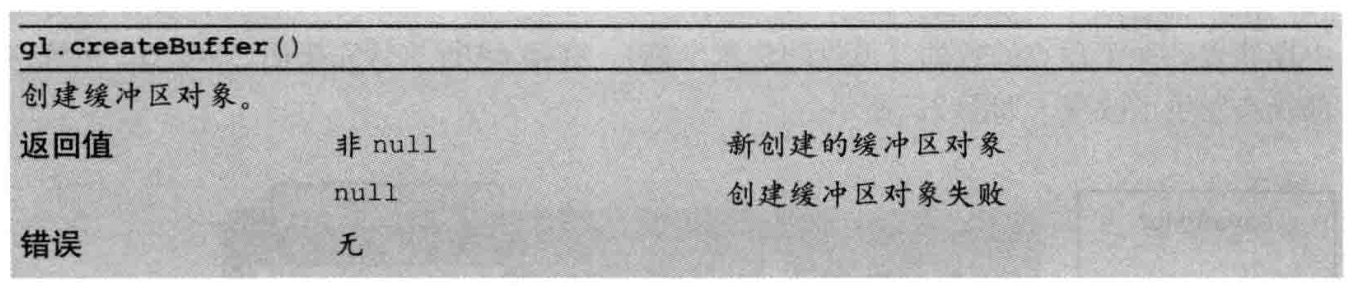### (2) 绑定缓冲区对象(gl.bindBuffer())

  // 将缓冲区对象绑定到目标
gl.bindBuffer(gl.ARRAY_BUFFER, vertexBuffer);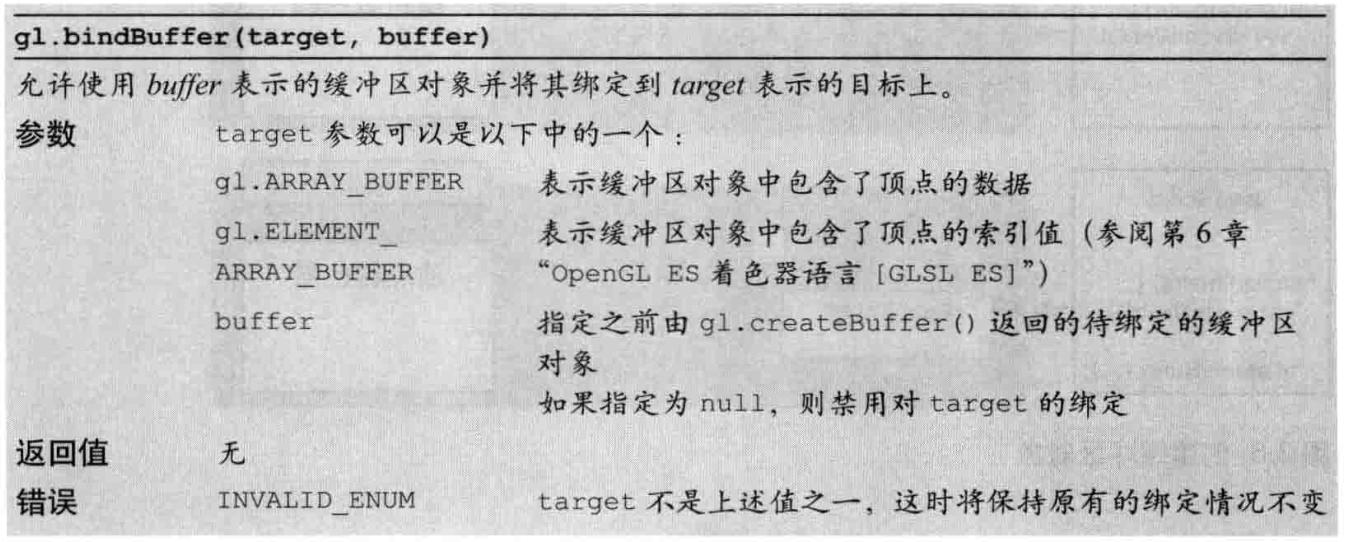### (3) 将数据写入缓冲区对象(gl.bufferData())

  // 向缓冲区对象写入数据
gl.bufferData(gl.ARRAY_BUFFER, vertices, gl.STATIC_DRAW);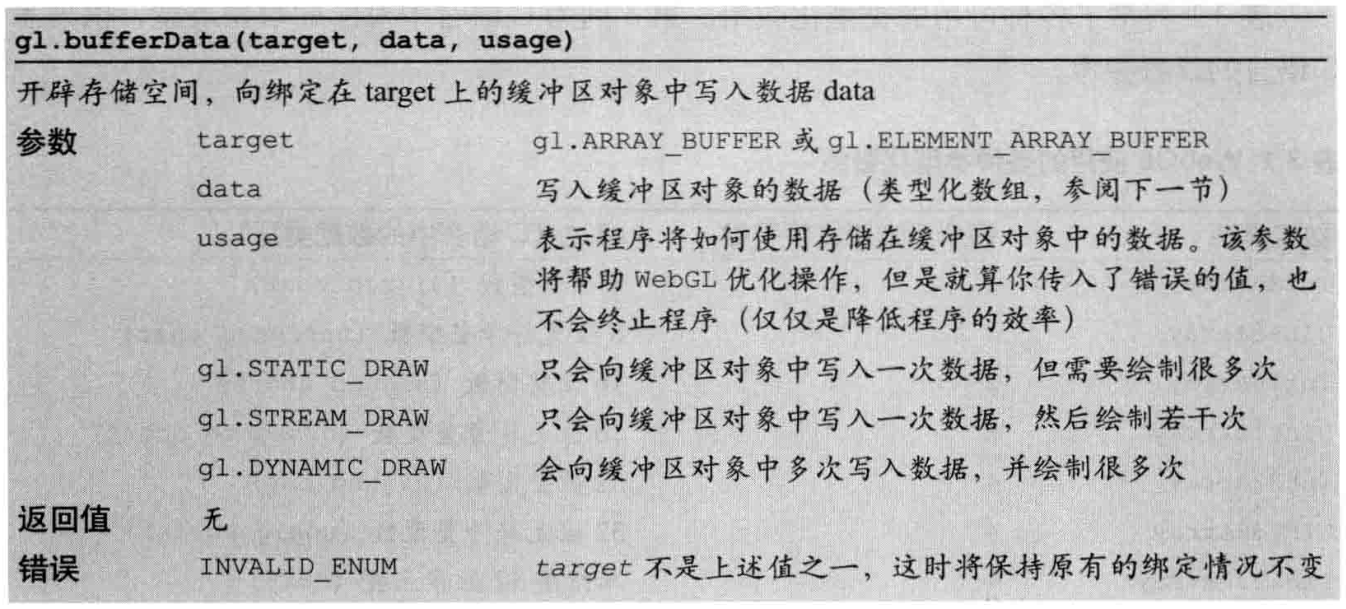### (4) 将缓冲区对象分配给attribute变量(gl.vertexAttribPointer())

  var a_Position = gl.getAttribLocation(gl.program, 'a_Position');
if (a_Position < 0) {
console.log('Failed to get the storage location of a_Position');
return -1;
}
// 将缓冲区对象分配给a_Position变量
gl.vertexAttribPointer(a_Position, 2, gl.FLOAT, false, 0, 0);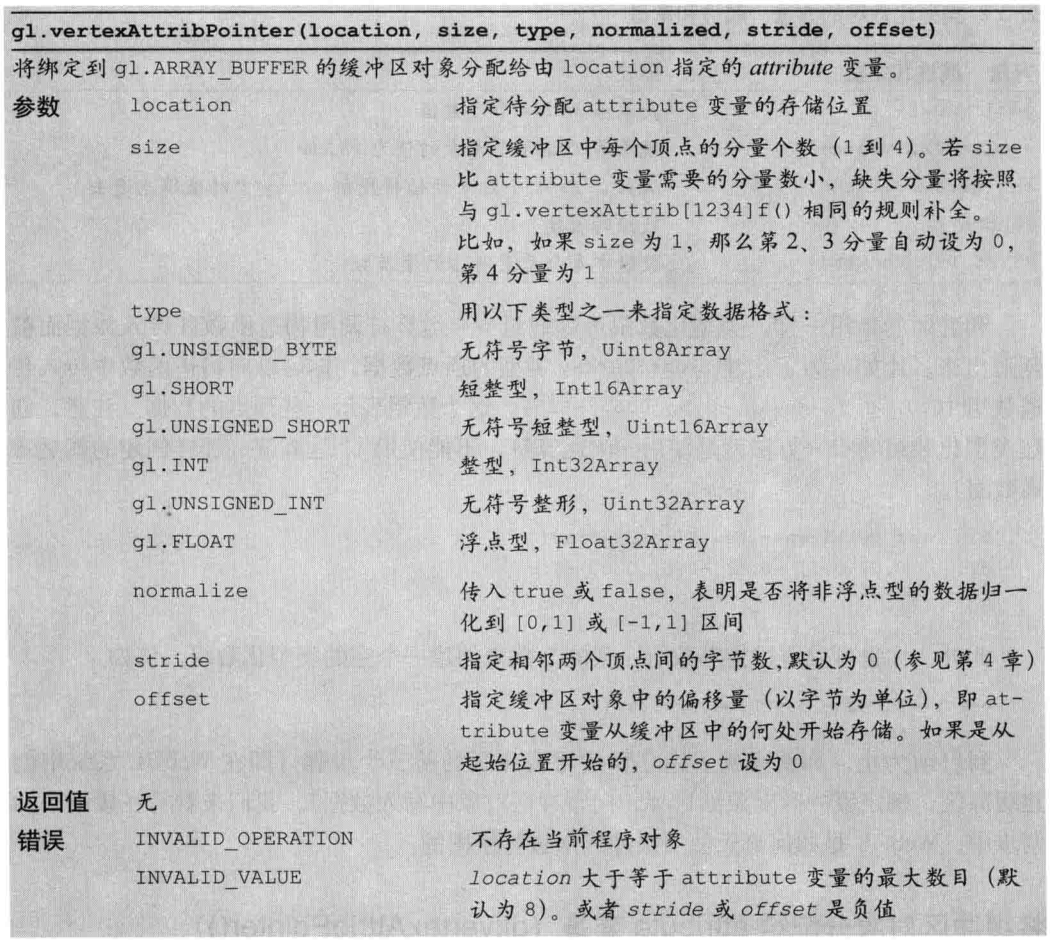### (5) 开启attribute变量(gl.enableVertexAttribArray())

  // 连接a_Position变量与分配给它的缓冲区对象
gl.enableVertexAttribArray(a_Position);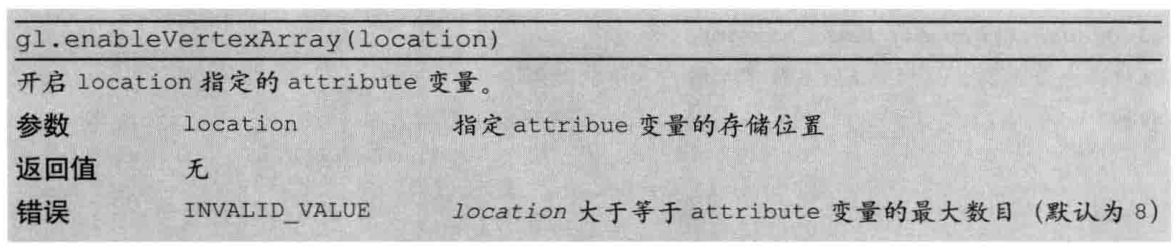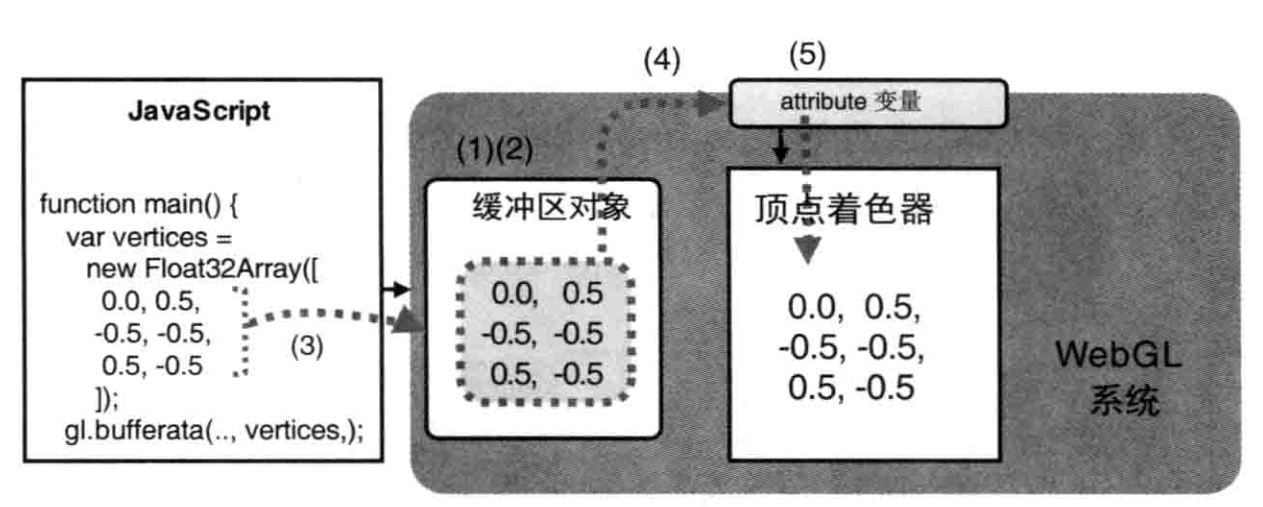## 4) 基本图形绘制

  // 绘制三角形
gl.drawArrays(gl.TRIANGLES, 0, 3);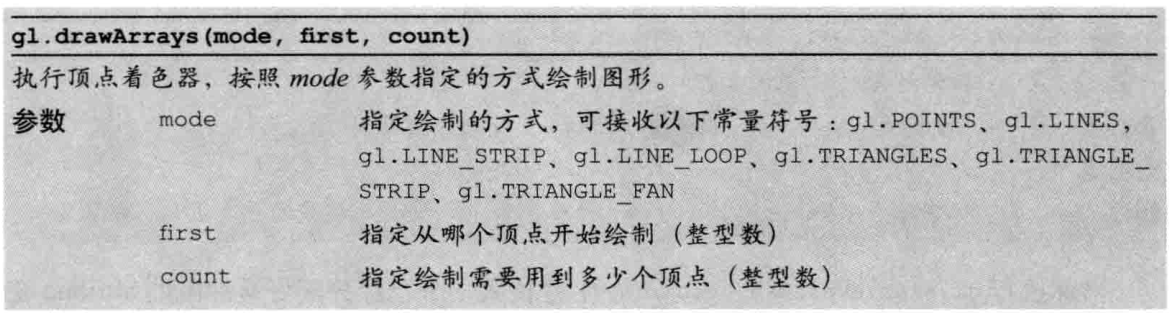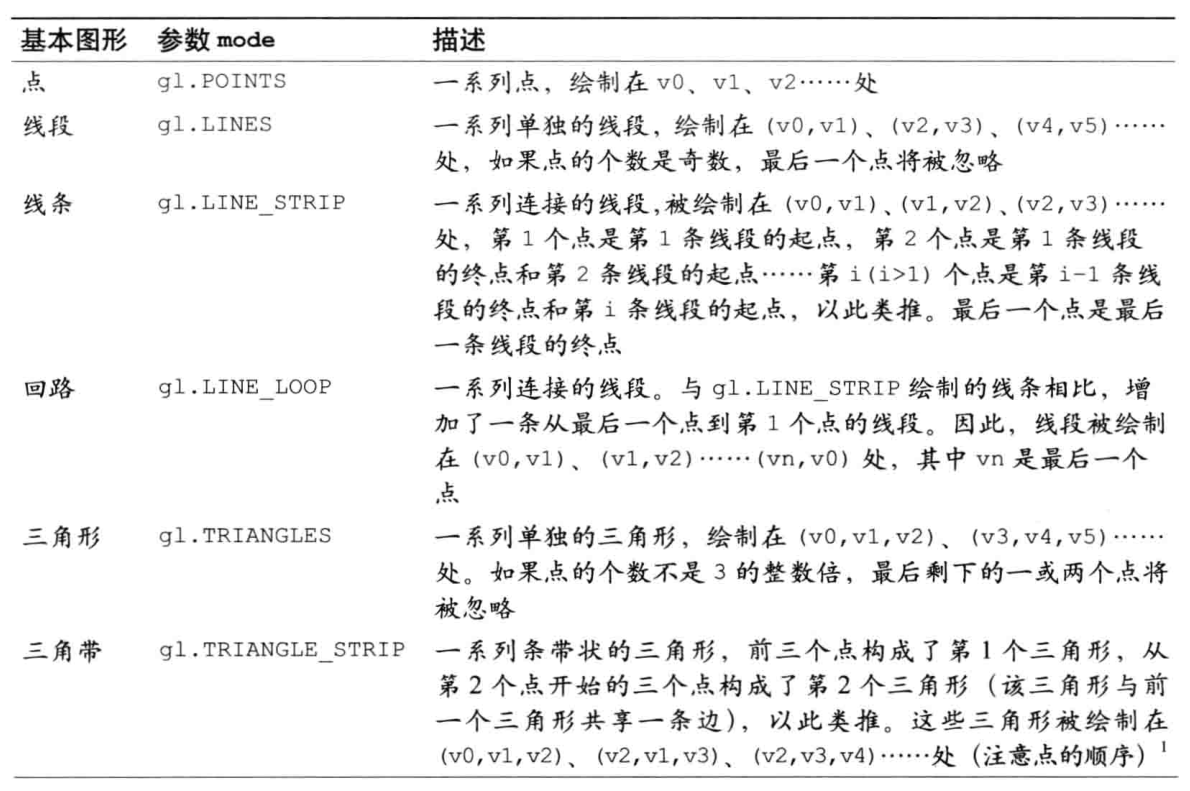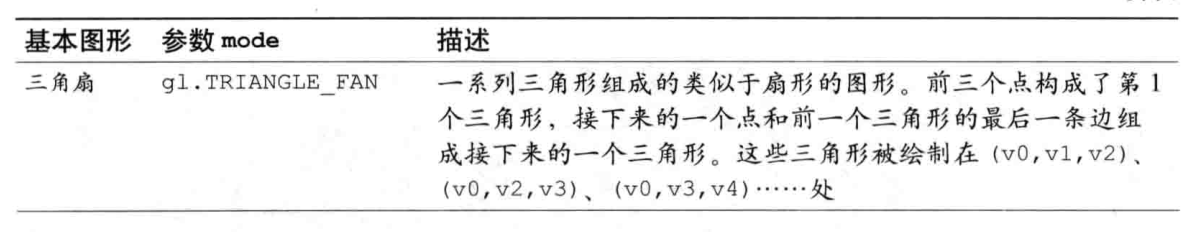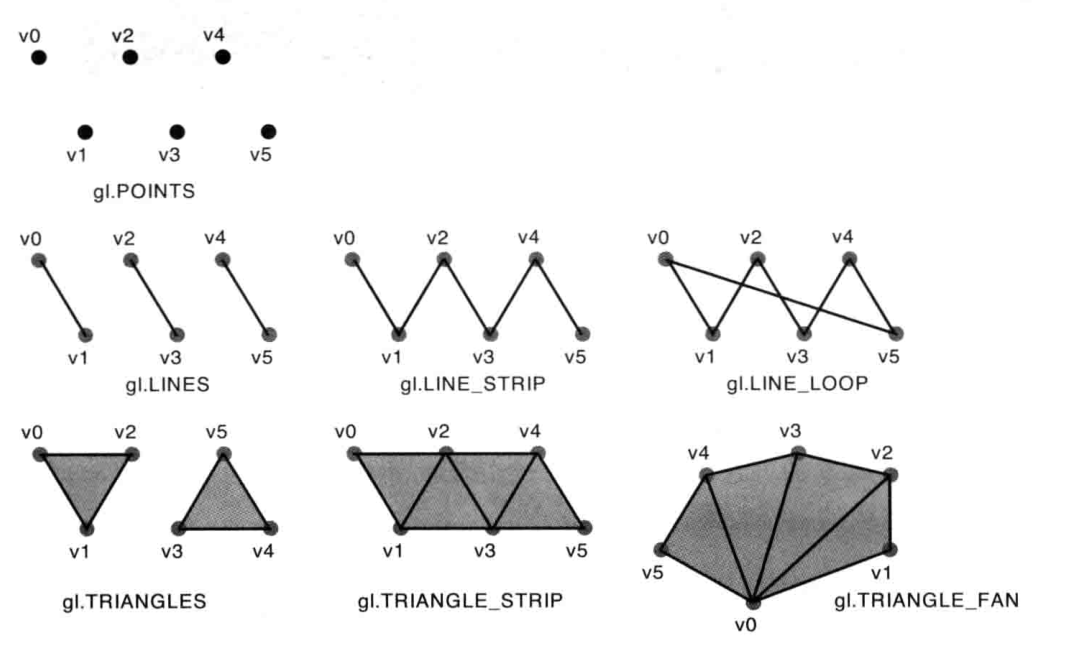# 3. 结果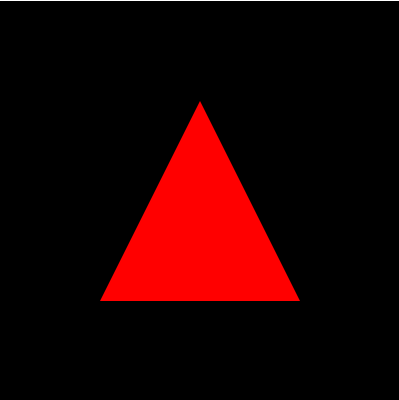# 4. 参考

posted @ 2019-09-04 11:09  charlee44  阅读(735)  评论(0编辑  收藏